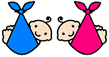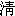Pregnancy and Baby Gender Chart using Chinese Lunar CalendarThe following Chinese Baby Gender Prediction Calendar Chart of ChingDynasty is the original chart published in 1972. Please read Where does this Chinese Baby Gender Prediction Chart come from? Some web sites have a little bit different charts. We don't know where they come from.

To use the chart, we have to use the Chinese age and Chinese Lunar calendar.

• Woman's age is the Chinese age
• Conception Month is the Chinese lunar month
• B stands for boy and blank stands for girl in the chart
• This is a statistic chart. B means the chance to be a boy more than a girl
 Age Woman Conception Lunar Month 1 2 3 4 5 6 7 8 9 10 11 12 18 B B B B B B B B B B 19 B B B B B B B 20 B B B B B B B B B 21 B 22 B B B B 23 B B B B B B B B 24 B B B B B 25 B B B B B B B B 26 B B B B 27 B B B B B B B 28 B B B B B B 29 B B B B B B 30 B B B 31 B B B 32 B B B 33 B B B B 34 B B B B 35 B B B B B B 36 B B B B B B B 37 B B B B B B B 38 B B B B B B 39 B B B B B B 40 B B B B B B 41 B B B B B B B 42 B B B B B B 43 B B B B B B B B 44 B B B B B B B 45 B B B B B B